## Unit 7 Study Guide: Variance Analysis

### 7a: Describe how flexible budgets are used to evaluate performance.

Managers use a flexible budget as a control measure to compare and evaluate actual costs to budgeted costs.

• Define a flexible budget.
• Why do managers adjust budgeted direct materials, direct labor, and overhead costs when actual production varies from budgeted production levels?

Review Flexible Budgets from Managerial Accounting.

### 7b: Explain how standard costs are established.

Managers use standard costs for planning and control purposes. Managers establish standard costs for direct materials, direct labor, and manufacturing overhead.

• Define and explain the difference between standard and budgeted costs?
• How do managers establish a standard price for direct materials?
• How do managers establish a standard quantity for direct materials?
• How do managers establish standard hours for direct labor?
• How do managers establish a standard rate for direct labor?
• How do managers establish a standard quantity for variable manufacturing overhead?
• How do managers establish a standard rate for variable manufacturing overhead?
• What is the difference between ideal and attainable standards?
• Where do managers find the information they use for establishing standard costs?

Review Standard Costs from Managerial Accounting.

### 7c: Calculate and analyze direct materials variances.

Managers should analyze the difference between actual direct material costs and budgeted direct material costs.

• How do managers calculate the difference between actual direct materials and budgeted direct materials for the cost of materials purchased?
• How do managers calculate the difference between actual direct materials and budgeted direct materials for the cost of materials used in production?
• List some reasons why direct material actual costs and what the costs should have been, according to the standards.

Review calculating and analyzing direct materials variances in Direct Materials Variance Analysis from Managerial Accounting.

Review Figure 10.4.

Figure 10.4 Direct Materials Variance Analysis for Jerry’s Ice Cream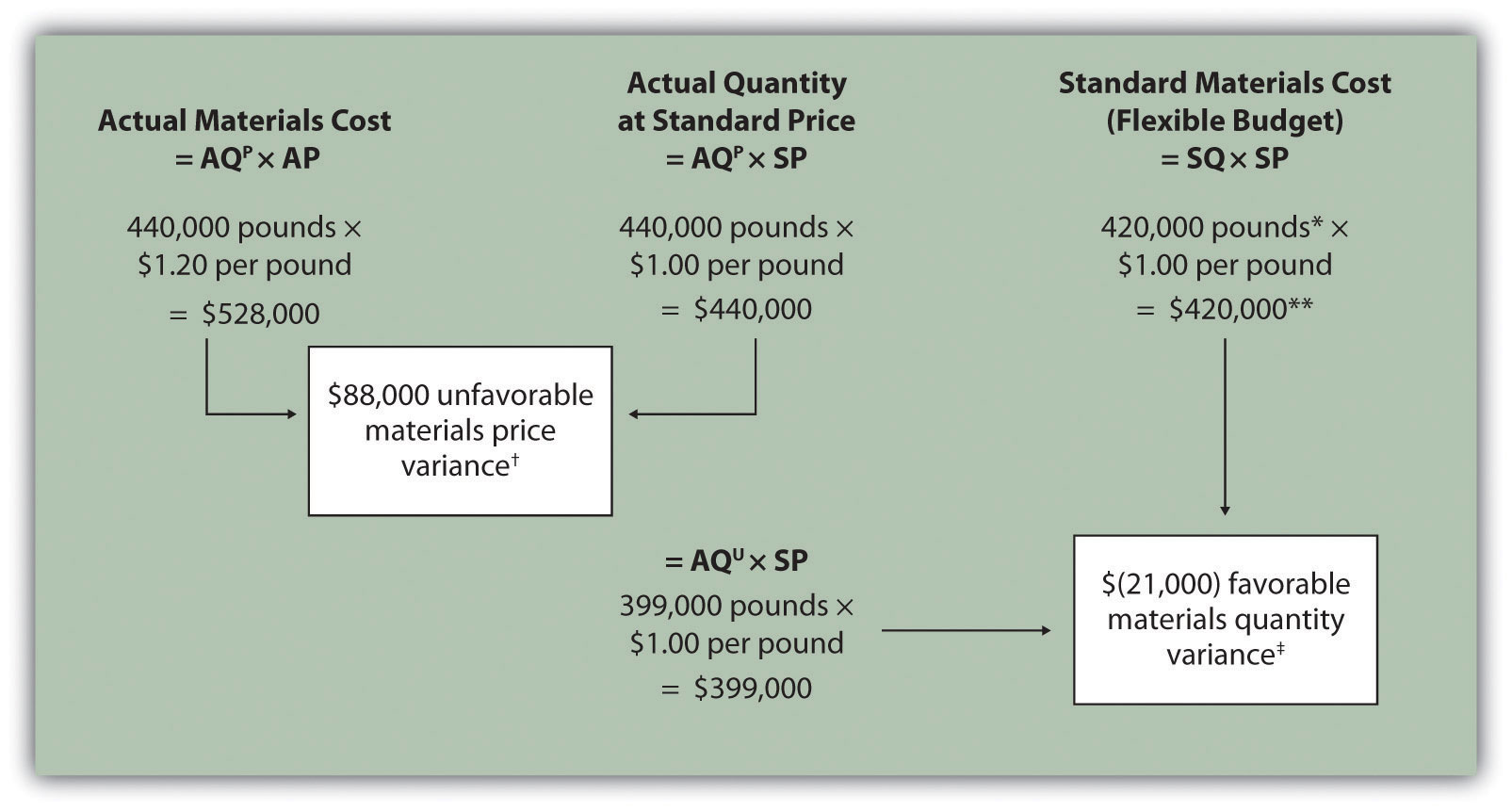### 7d: Calculate and analyze direct labor variances.

Managers should analyze the difference between actual direct labor costs and budgeted direct labor costs.

• How do managers calculate the difference between actual direct labor and budgeted direct labor for the hourly direct labor rate.
• How do managers calculate the difference between actual direct labor and budgeted direct labor for the number of hours worked.
• List some reasons why variances occur in direct labor actual costs and what the costs should have been, according to the standards.

Review calculating and analyzing direct labor variance in Direct Labor Variance Analysis from Managerial Accounting.

Review Figure 10.6.

Figure 10.6 Direct Labor Variance Analysis for Jerry’s Ice Cream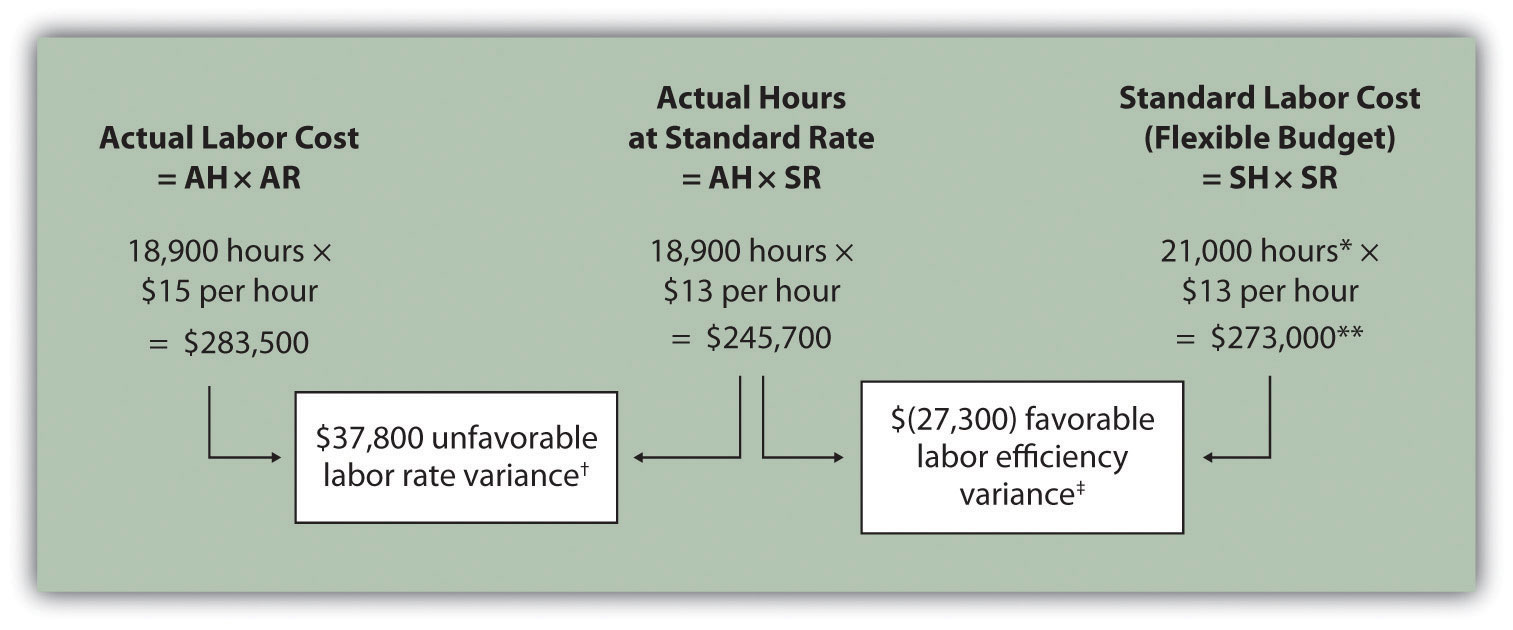### 7e: Calculate and analyze variable manufacturing overhead variances.

Managers should analyze the difference between actual overhead costs and budgeted overhead costs.

• How do managers calculate the difference between actual overhead and budgeted overhead for the amount spent?
• How do managers calculate the difference between actual overhead and budgeted overhead for the number of direct labour hours worked.
• List some reasons why variances occur in actual overhead costs and what the costs should have been, according to the standards.

Review calculating and analyzing manufacturing overhead variance in Variable Manufacturing Overhead Variance Analysis from Managerial Accounting.

Review Figure 10.8.

Figure 10.8 Variable Manufacturing Overhead Variance Analysis for Jerry’s Ice Cream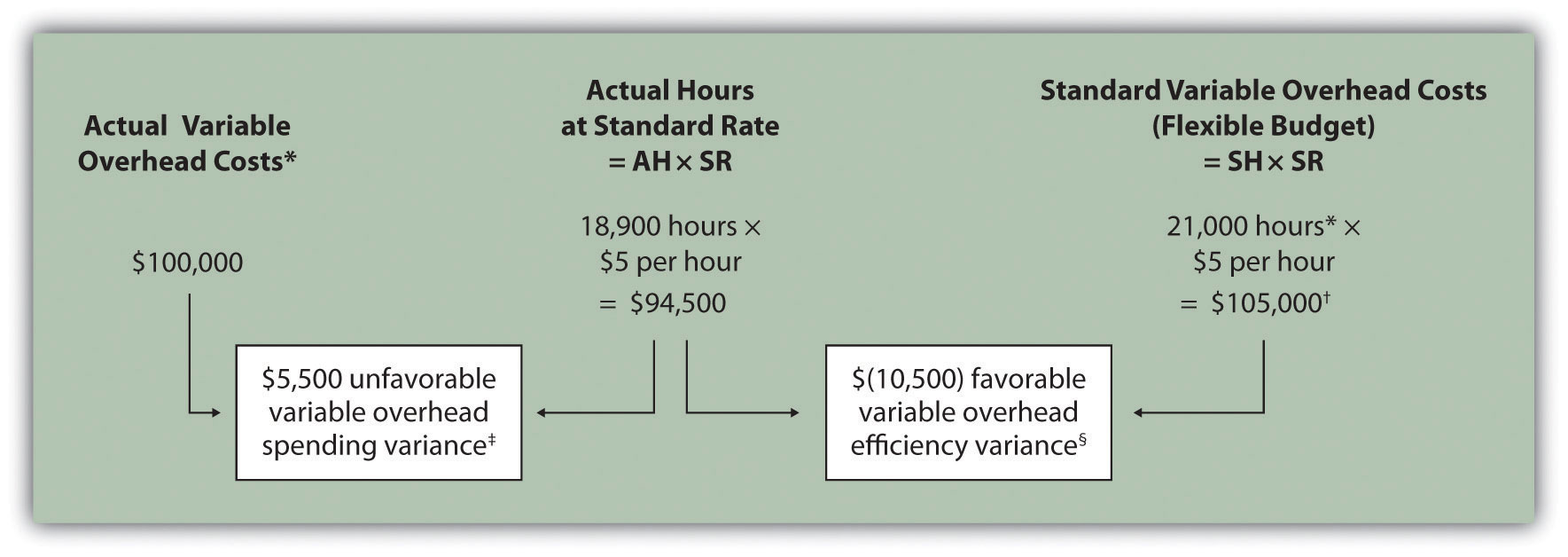### 7f: Determine which variances to investigate.

Most companies or organizations lack the time or resources to investigate every single variance.

• Define management by exception.
• List some reasons why managers should investigate favorable and unfavorable variances.

Review Determining Which Cost Variances to Investigate from Managerial Accounting.

Review Figure 10.14.

Figure 10.14 Comparison of Variable and Fixed Manufacturing Overhead Variance Analysis for Jerry’s Ice Cream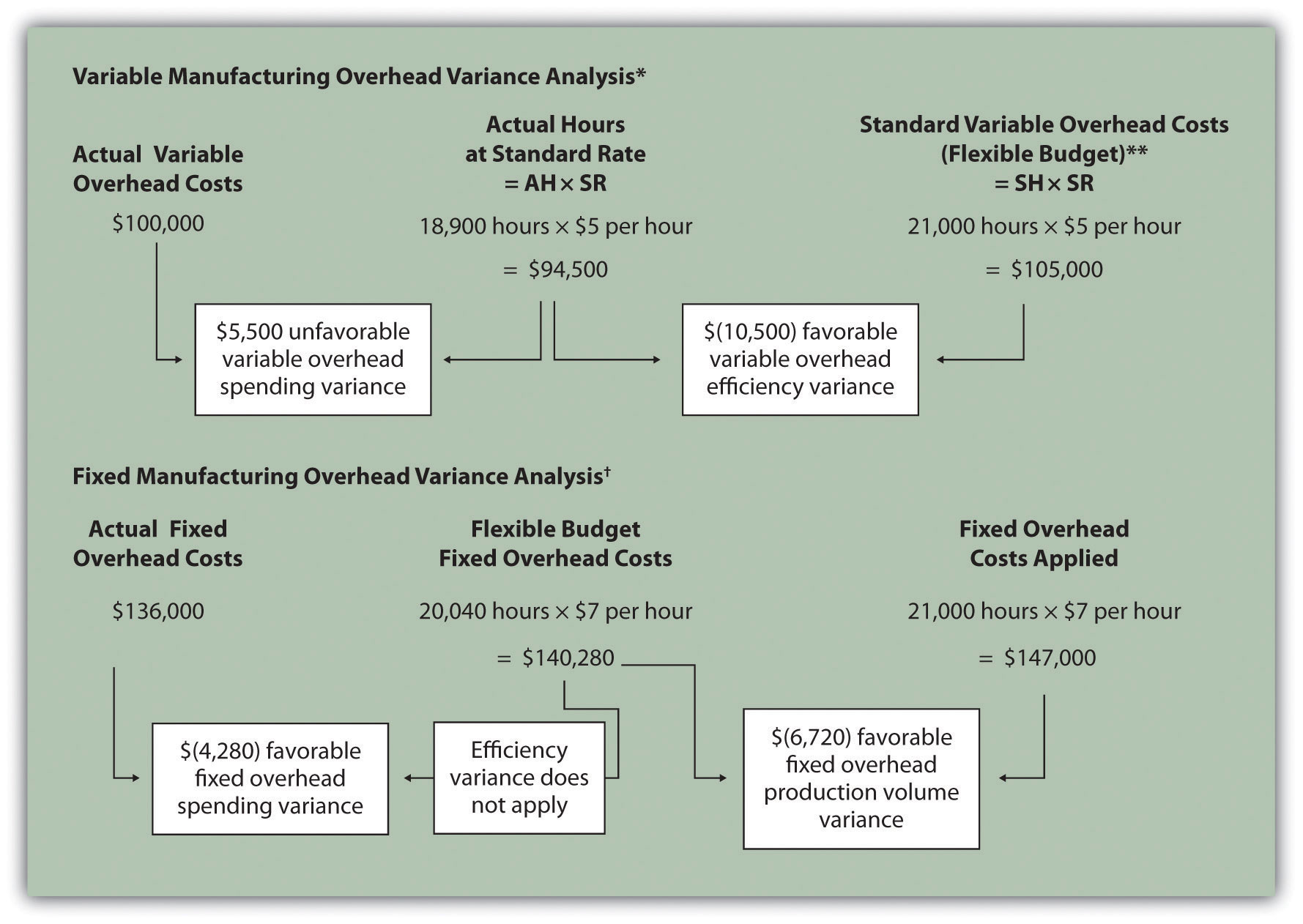### 7g: Explain how to use cost variance analysis with activity-based costing.

Managers integrate activity-based costing methods with cost variance analysis to further understand the cause of variances.

• Define variable overhead spending and efficiency variances.
• How can managers use multiple cost pools and cost drivers to help identify the source of variances?
• How do managers calculate variable overhead spending and efficiency variances?

Review Using Variance Analysis with Activity-Based Costing from Managerial Accounting.

Review Figure 10.11.

Figure 10.11 Variable Overhead Variance Analysis for Jerry’s Ice Cream Using Activity-Based Costing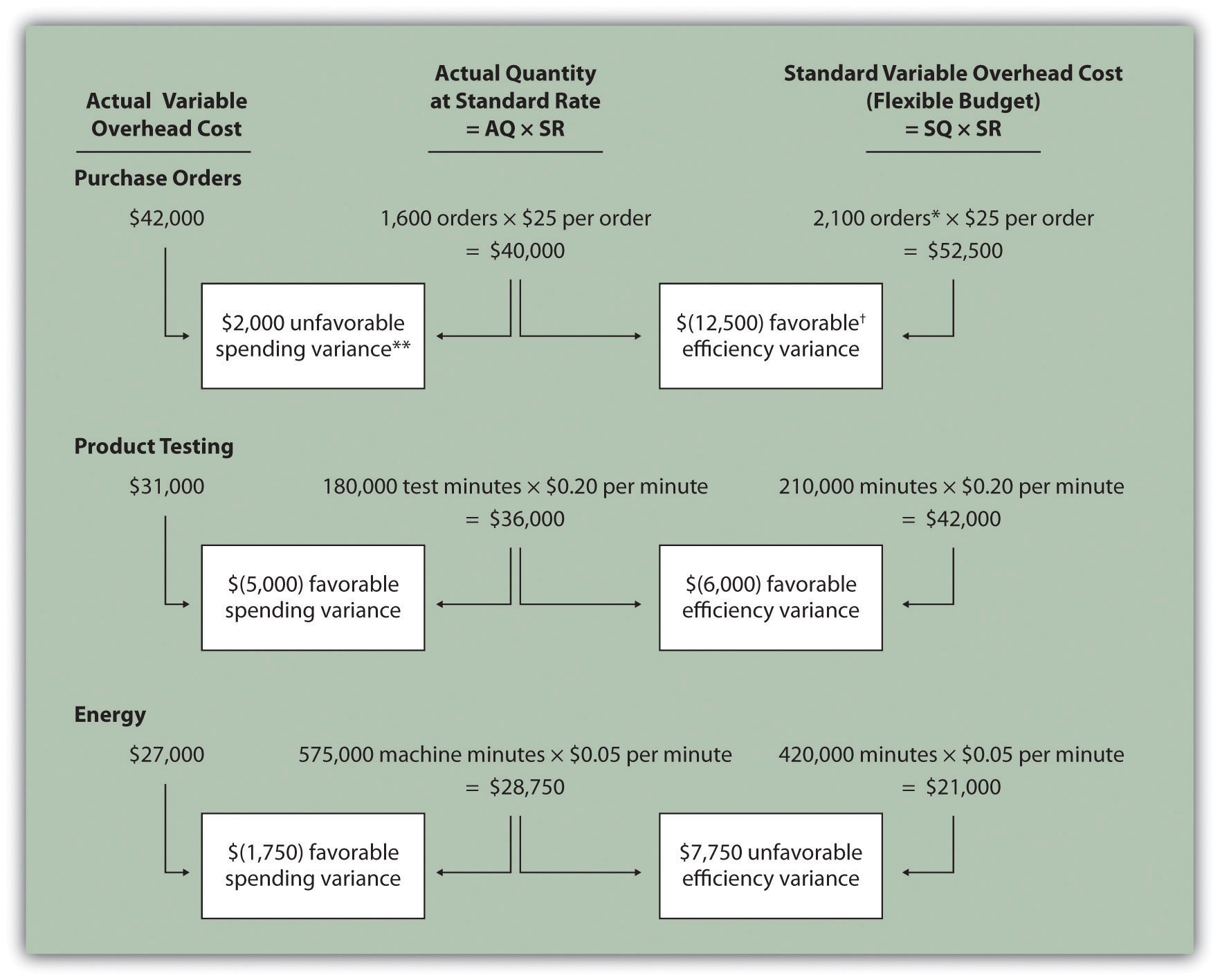### 7h: Calculate and analyze fixed manufacturing overhead variances.

Managers should analyze the differences among actual fixed overhead costs, budgeted fixed overhead costs, and applied fixed overhead costs.

• How do managers calculate the difference between actual fixed overhead and budgeted fixed overhead for the amount spent?
• How do managers calculate the difference between actual fixed overhead and applied fixed overhead for the amount spent?
• What is the difference between fixed and variable overhead variances?

Review Fixed Manufacturing Overhead Variance Analysis from Managerial Accounting.

Review fixed overhead variances in Figure 10.12, Figure 10.13, and Figure 10.14.

Figure 10.12 Fixed Manufacturing Overhead Information for Jerry’s Ice Cream.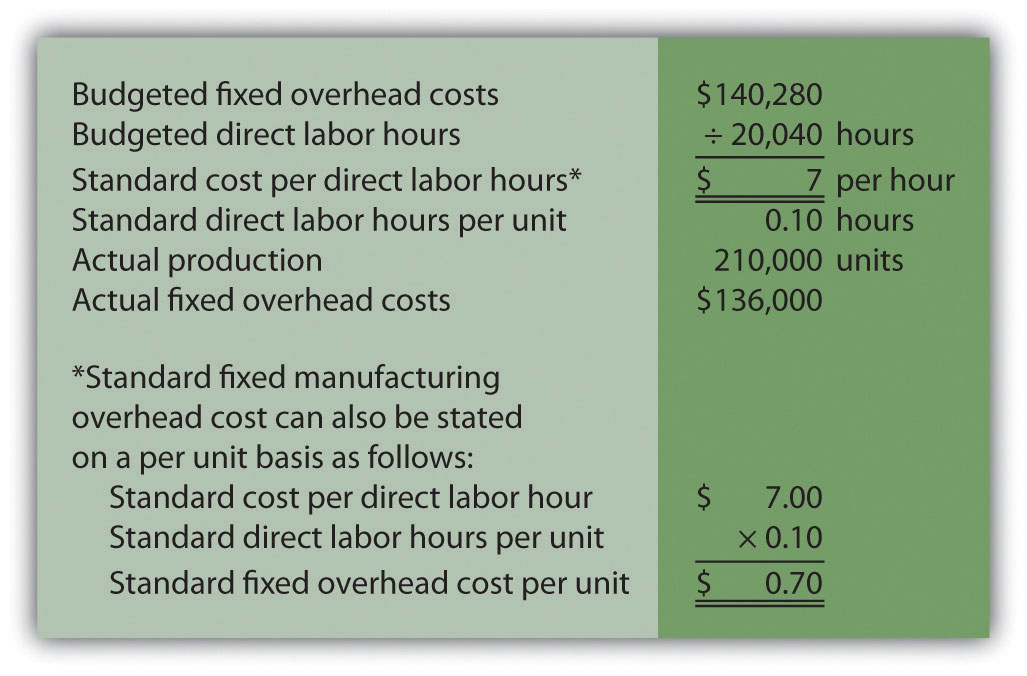Figure 10.13 Fixed Manufacturing Overhead Variance Analysis for Jerry’s Ice Cream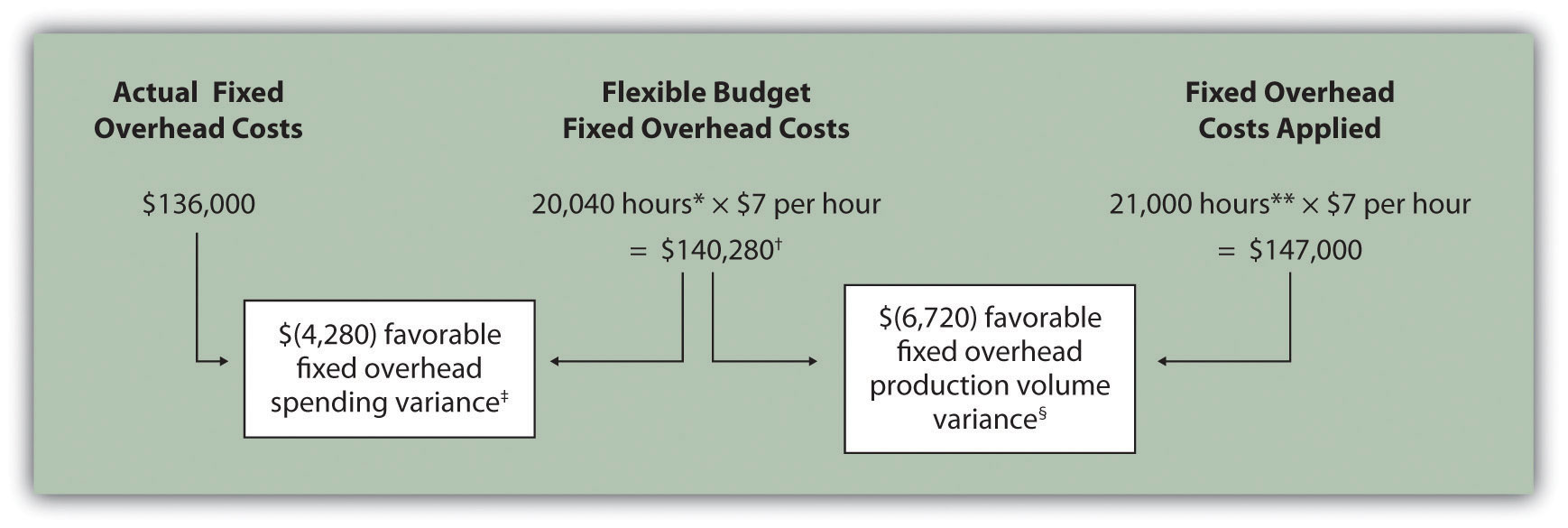Figure 10.14 Comparison of Variable and Fixed Manufacturing Overhead Variance Analysis for Jerry’s Ice Cream### Unit 7 Vocabulary

• Attainable standards
• Favorable variance
• Fixed overhead production volume variance
• Flexible budget
• Ideal standards
• Labor efficiency variance
• Labor rate variance
• Management by exception
• Materials price variance
• Materials quantity variance
• Standard cost
• Standard hours
• Standard price
• Standard quantity for direct materials
• Standard quantity for variable manufacturing overhead
• Unfavorable variance